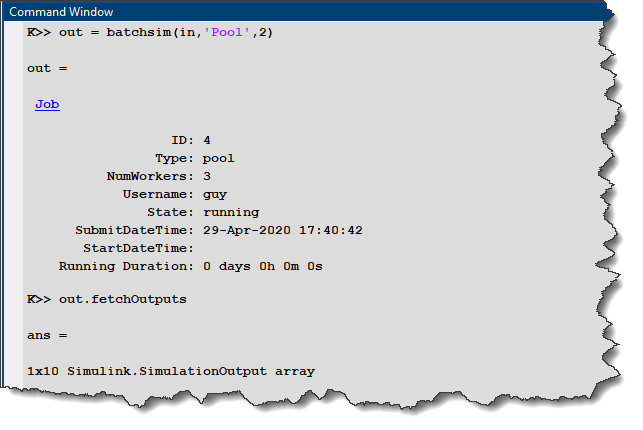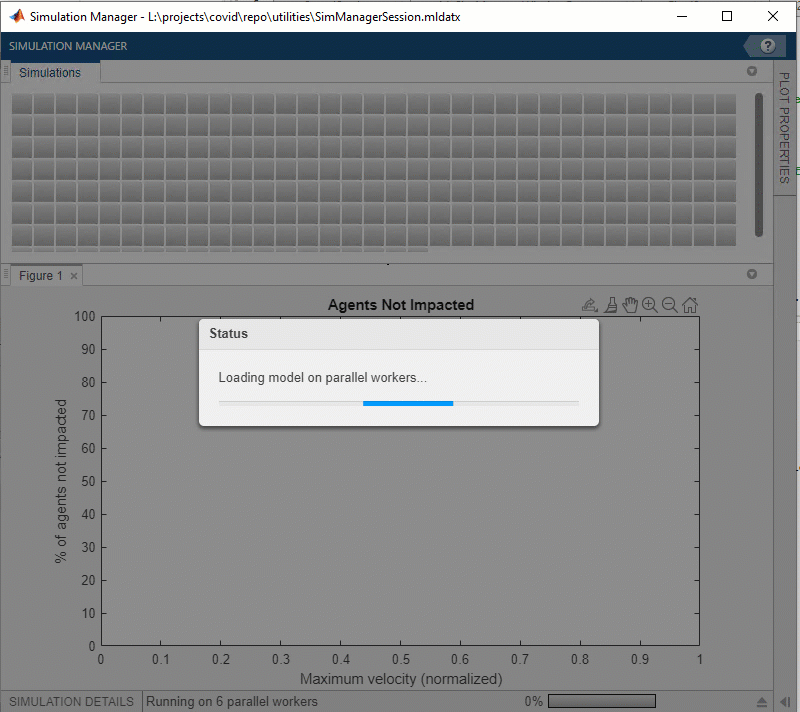# COVID-19: Using Parallel Simulations to Study the Infection Spread

In a previous post, I introduced a model simulating the exponential spread of a phenomenon like COVID-19. With more and more talks in the news about deconfinement plans, I thought it would be interesting to run multiple simulations with different deconfinement scenarios and observe the potential outcomes.

Here is one example result shown in the Simulation Manager: I ran 250 simulations where I varied the initial speed of the agents. This parameter is analogous to how well people respect confinement rules. You can see that for the extreme cases, the results are straightforward: with a slow velocity, where everybody is in confinement, almost nobody gets infected. At the opposite, with large velocity, everybody gets infected. What is most interesting is in the middle. As you can see, for a range of initial velocity, the outcome is difficult to predict.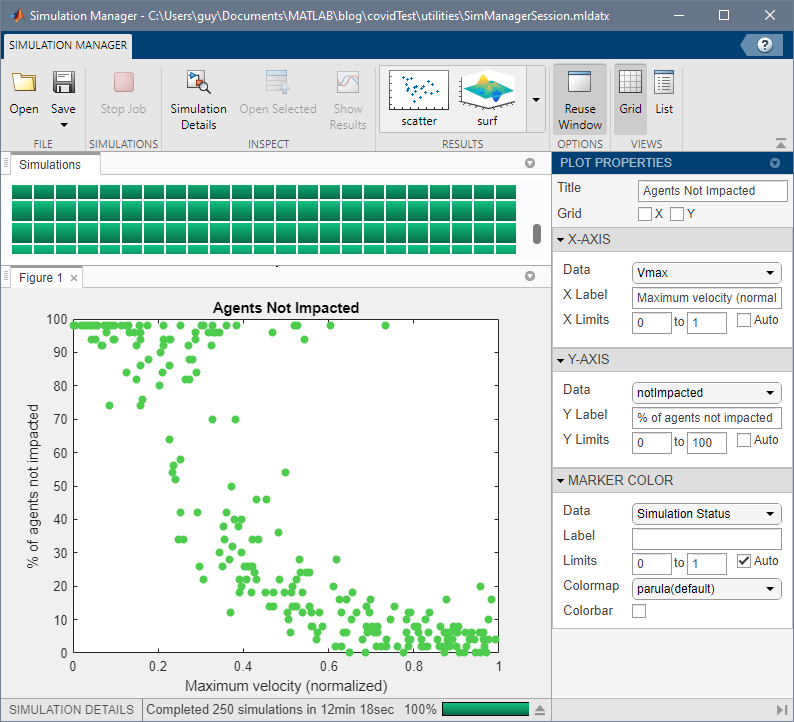Let's see how I used parsim and the Simulation Manager to run those simulations utilizing all the cores on my computer and produce the above image.

If you are not familiar with parsim, I recommend visiting this earlier post where I introduced this function.

Managing Simulation Data

As you probably know, there are multiple ways to specify the data needed by a model. With options like Data Dictionary, Model workspace, base workspace, and Simulink.SimulationInput object, it's understandable to be confused about what to choose. For this project, I could have probably used any combinations of those methods and get the results I want. Here is what I decided to use, and why.

To begin, I decided to store a set of default values for all the parameters needed by the model in the Model Workspace. While developing the model, it allows me to update the diagram without the need for any specific variables defined in the base workspace ("Keep your model in an updatable state as much as possible" is one of the first and main advice I repeat when teaching Simulink).

As you probably know, the Model Workspace has four options for the data source: The model file, a MAT-file, a MATLAB file (a MATLAB script), and MATLAB code. I chose a MATLAB script.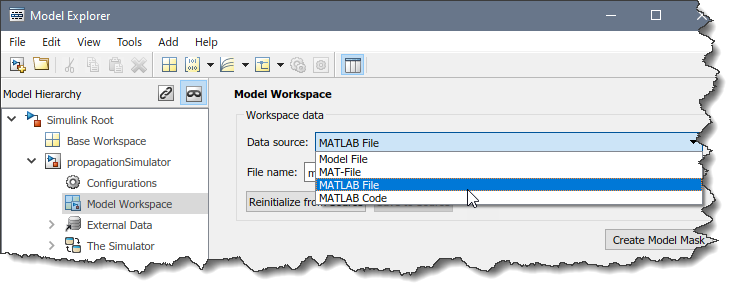When the time comes to simulate the model using parsim, I can specify the parameters to be tuned using Simulink.SimulationInput objects and the setVariable method.

Here is my code preparing the array of simulation input objects to be passed to parsim: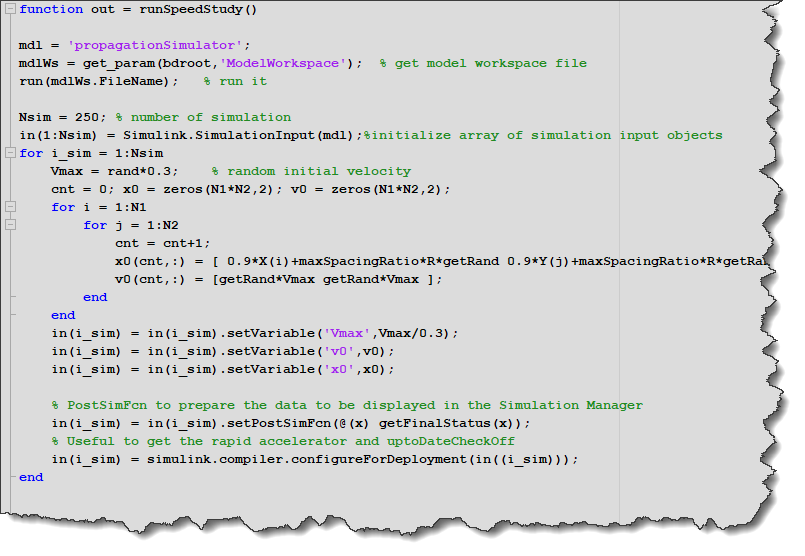I hope this becomes clearer why I defined the model workspace in a MATLAB script: I need values from the model workspace to create the simulation input objects. In the project, I have a fixed number of agents with default initial positions. In the simulation input objects, what I do is modifying their initial position with a random disturbance and assigning them initial velocities.

Post Simulation Function

In the above screenshot, you probably noticed that I used the setPostSimFcn method of the simulation input object and I pass to it a handle to the function I want to be executed on each worker after the simulation is completed.

This function will receive as input the Simulink.SimulationOutput object produced by the model, and is supposed to produce the Simulink.SimulationOutput object that parsim will return for this simulation. This can typically be used in two ways:

• To do post processing of the logged data and add results to the original simulation output object
• To do post processing of the logged data and replace the original simulation output object

As you can imagine, this second option becomes useful when running a large amount of simulations that each log lots of data. In my case, here is what my post-simulation function looks like: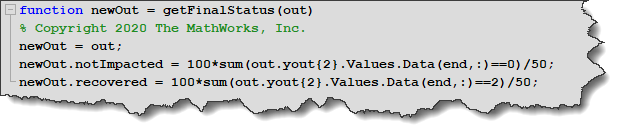In this code, I compute how many agents, at the last time step of the simulation, have never been infected, and how many have been infected and are now immune.

Visualizing Results in the Simulation Manager

With all that set up, I can visualize the results as the individual simulations complete on the parallel workers in the simulation manager. Since I added the number of agents never infected to the simulation output object, I can create a scatter plot that will display this number versus the agents' initial velocity.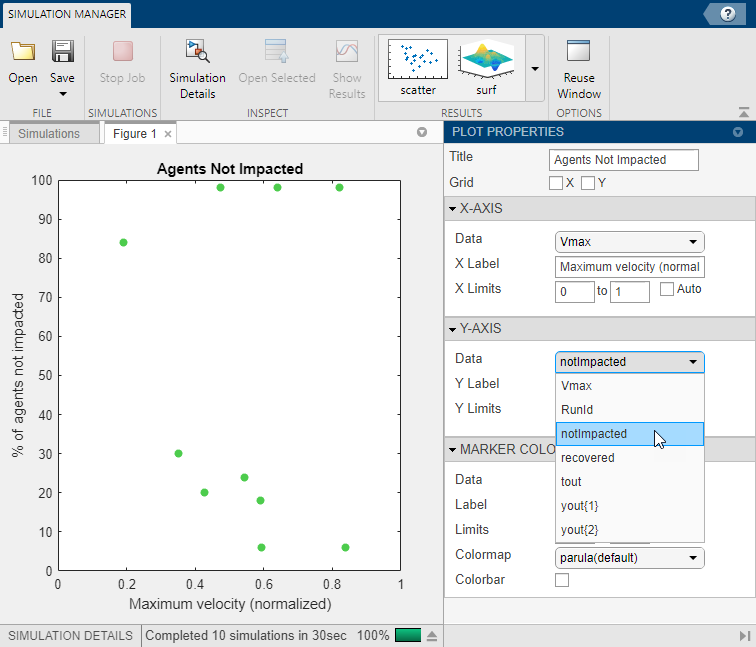Simulating in Parallel

At this point, it's time to run those simulations. I would like to highlight 3 possible ways to do this. You can read more on multiple simulations workflows here.

Option 1 - parsim: By default, parsim is a blocking function; it will return the command prompt only when all the simulations are completed.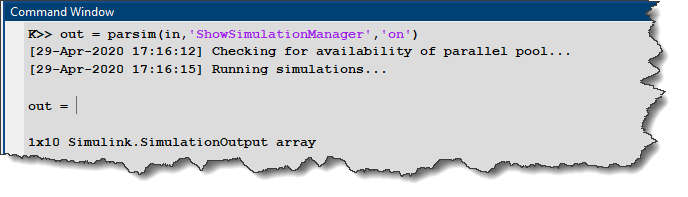Option 2 - parsim in background: Parsim has an option to run in the background. With this option, parsim will return the MATLAB prompt immediately so you can do other work in MATLAB while the parallel workers are running the simulations. In this case, parsim returns an array of Simulink.Simulation.Futures. When the state property of those future objects changes to "finished", it is possible to use the fetchOutput method to retrieve the simulation output objects.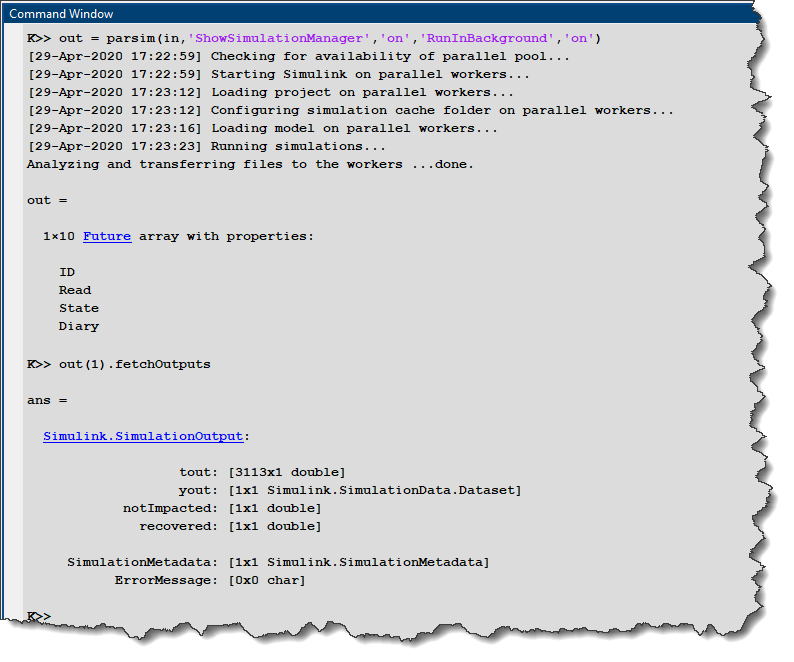Option 3 - Batchsim: With batchsim, we are taking one step further and let everything be managed on the parallel cluster. In this case, one parallel worker becomes the head worker and is responsible to dispatch simulations to the other parallel workers and collect results. It requires one more worker, but it has the advantage that you can shutdown MATLAB and reconnect later to get the job and fetch the results.